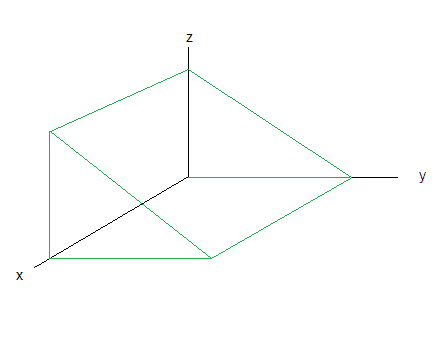# Maximizing volume

## Homework Statement

Canada Post accepts international parcels whose (Length+Girth) is less than or equal to 2 meters, and Length is less than or equal to 1 meter. Girth is defined as the cross section. We wish to ship a parcel of the shape of a triangular prism of length l meters. The cross section is a right triangle with catheti of lengths a and b meters. Assume the package walls are thin. What is the maximal volume of a parcel?

## Homework Equations

Let
$$leg_1=a=y, leg_2=b=z, length=x$$
I provided a drawing via paint for you to envision my take on the problemHence,
$$V(x, y, z)=\frac{1}{2}xyz$$
Boundaries:
$$(x+y+z+\sqrt{x^2+y^2})\leq{2}, x\leq{1}$$

## The Attempt at a Solution

$$\nabla{f}(x, y, z)=\frac{1}{2}(yzi+xzj+xyk)$$
and hence there exists a critical point at (0, 0, 0).

Next, I get partially lost. Should I be finding second partial derivatives of the boundary and then evaluating the Hessian matrix to determine extremes on the boundary?

Ray Vickson
Homework Helper
Dearly Missed

## Homework Statement

Canada Post accepts international parcels whose (Length+Girth) is less than or equal to 2 meters, and Length is less than or equal to 1 meter. Girth is defined as the cross section. We wish to ship a parcel of the shape of a triangular prism of length l meters. The cross section is a right triangle with catheti of lengths a and b meters. Assume the package walls are thin. What is the maximal volume of a parcel?

## Homework Equations

Let
$$leg_1=a=y, leg_2=b=z, length=x$$
I provided a drawing via paint for you to envision my take on the problemHence,
$$V(x, y, z)=\frac{1}{2}xyz$$
Boundaries:
$$(x+y+z+\sqrt{x^2+y^2})\leq{2}, x\leq{1}$$

## The Attempt at a Solution

$$\nabla{f}(x, y, z)=\frac{1}{2}(yzi+xzj+xyk)$$
and hence there exists a critical point at (0, 0, 0).

Next, I get partially lost. Should I be finding second partial derivatives of the boundary and then evaluating the Hessian matrix to determine extremes on the boundary?

Critical points of V are irrelevant in this problem, due to the presence of constraints. Here is a simpler example: what is the maximum of f(x) =x^2, subject to 0 <= x <= 1? Obviously, the max is at x = 1, but the derivative of f is 2 at that point, not zero.

RGV

hmm, and when I tried finding critical points within the bounded portion the gradient told me there were not. It doesn't seem logical to just start plugging numbers in, as there are three variables that depend on girth.

Ray Vickson
Homework Helper
Dearly Missed
hmm, and when I tried finding critical points within the bounded portion the gradient told me there were not. It doesn't seem logical to just start plugging numbers in, as there are three variables that depend on girth.

You have forgotten three very important restrictions: x >= 0, y >= 0, z >= 0. If you were to submit your problem to a computer package, but without these restrictions, you would get a nonsensical solution: you can let x and y go to -infinity, and fix z = 1. The both of your written restrictions are satisfied, and V --> + infinity (which is certainly as much of a maximum as you could want!).

max V(x,y,z)
subject to g(x,y,z) <= 2, x <= 1 and x, y, z >= 0.
(Here, V and g are the functions you wrote.)

You can solve this problem using the Karush-Kuhn-Tucker conditions (essentially, the Lagrangian conditions extended to inequality constraints). If all you want is a numerical solution, and don't care how you get it, you can use the EXCEL Solver tool to get a solution in an EXCEL spreadsheet. Other spreadsheets (including free, open source ones) also have solver tools.

RGV

Okay, I have had a bit more experience with Lagrange multipliers, hence here is my next attempt.
The maximum must occur on the boundary of,
$$(x+y+z+\sqrt{z^2+y^2})={2}$$
Hence, let
$$L=0.5xyz+\lambda{(}x+y+z+\sqrt{y^2+z^2-2})$$
The critical points of L are determined via
$$L_1=0.5yz+\lambda$$
$$L_2=0.5xz+\lambda{+}\frac{2y}{\sqrt{y^2+z^2}}$$
$$L_3=0.5xy+\lambda{+}\frac{2z}{\sqrt{y^2+z^2}}$$
$$L_4=x+y+z+\sqrt{x^2+y^2}-2$$
Is there any easy way to compute the solutions without using computational software?

I guess the next step would be to solve the system of equations for the four unknowns, since there are four equations.

ALso was informed that there was an easier method to solving this question.

Last edited: